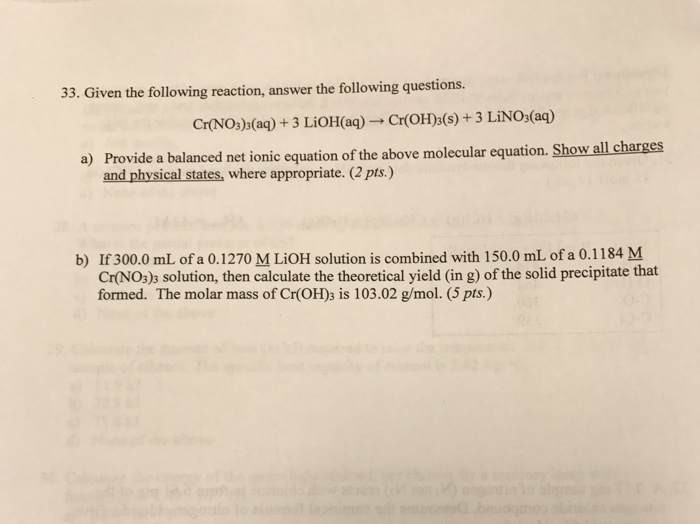# Question & Answer: Given the following reaction, answer the following questions. Cr(NO_3)_3(aq) + 3 LiOH(aq)…..Given the following reaction, answer the following questions. Cr(NO_3)_3(aq) + 3 LiOH(aq) Rightarrow Cr(OH)_3(s) + 3 LiNO_3(aq) a) Provide a balanced net ionic equation of the above molecular equation. Show all charges and physical states, where appropriate. b) If 300.0 mL of a 0.1270 M LiOH solution is combined with 150.0 mL of a 0.1184 M Cr(NO_3)_3 solution, then calculate the theoretical yield (in g) of the solid precipitate that formed. The molar mass of Cr(OH)_3 is 103.02 g/mol.

a) net ionic equation as follows

Cr+3(aq) + 3OH(aq)    ————-> Cr(OH)3(s)

Don't use plagiarized sources. Get Your Custom Essay on
Question & Answer: Given the following reaction, answer the following questions. Cr(NO_3)_3(aq) + 3 LiOH(aq)…..
GET AN ESSAY WRITTEN FOR YOU FROM AS LOW AS \$13/PAGE

b) moles of LiOH = (0.1270)(300/1000) = 0.0381

moles of Cr(OH)3 = 0.1184 (150/1000) = 0.0178

so LiOH is limiting reagent.

3 mole LiOH gives 1 mole Cr(OH)3

0.0381 moles LiOH gives (0.0381 x 1/3) = 0.0127 moles

convert moles to mass

mass = moles x molar mass = 0.0127 x 103.02 = 1.31 g

mass of Cr(OH)3 formed = 1.31 g